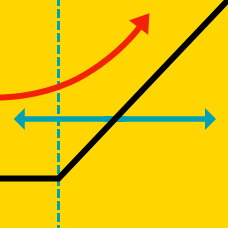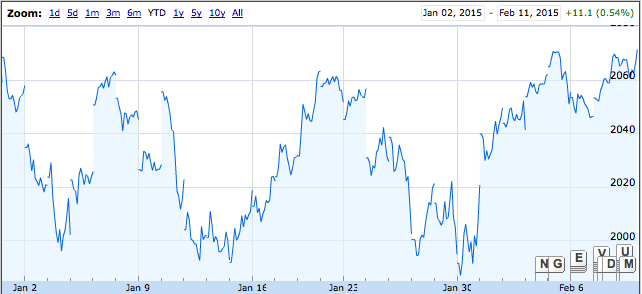Quantitative Finance

# Options: Level 2-3 ChallengesHow is historical trading-day volatility measured?

Step 1:
A) Calculate the absolute change in closing prices as the data set.
B) Calculate the percentage change in closing prices as the data set.

Step 2:
C) Calculate the standard deviation of the data set.
D) Calculate the variance of the data set.

Step 3:
E) Multiply it by $\sqrt{ 252}$.
F) Multiply it by $\sqrt{365}$.

When would you want to early exercise an American call?

The stock is trading at $20. The European call on the$10 strike expiring in 1 months is actively trading at $9. What trade do you want to execute? The stock is currently trading at$30. What is the maximum price of the Put on the \$20 strike, expiring in 1 year?

When would you early exercise an American put option on a stock that doesn't pay dividends?

×